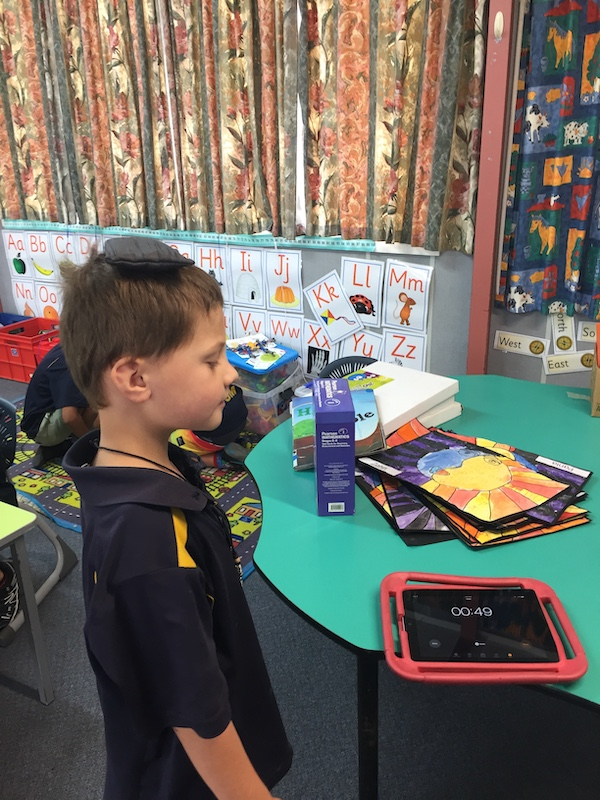# Geometry and measurement

### Geometry and measurement learning involves lots of fun, hands-on, real-life learning activities.  Here are some of the learning that Room 14 took part in.The window is about 5 12 pens wideMater is about 6 pens long

When we measure something by counting whole numbers of units we are learning about using non-standard measurements.  We learn that you can measure with lots of things e.g. paper clips, cubes, counters, pens, straws, blocks. The rules of using non-standard measurements are:

• The object (e.g. cubes) must be the same size.
• You must measure from edge to edge.
• There can be no overlaps or gaps.
• When we use non-standard measurement we use the word about e.g. the pen is about 7 cubes long, because non-standard measurements are often not exact.Yes I did it now to count how many cubes I used

#### Non-Standard Measurement

I measured my watch using beads.  It was 16 beads long. Travis
I measured the cupboard with straws.  It was 15 straws long. Kiara
I used the straws to measure how wide the classroom is.  It was 34 straws wide. Clara
I measured the table using cubes.  I measured the length and width. The length was 75 cubes and the width was 44 cubes. Sam
I used the 48 marbles to measure the width of the table.  Declan
I measured the width of the classroom using pens.  It was 57 pens wide. Tom
Some of us tried to measure the length of the classroom using cubes.  We found two problems. One was that we ran out of cubes. The other was that we were using so many cubes it was hard to count.  Maybe we should of used a bigger unit. AnnabelleHere are some things we can use to make non standard measurementsHard at work measuring the length of the tableThis cushion is 17 counters long